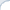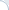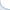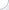One well-studied NLP task is answering analogy questions. For example: to whom the word woman relates in a similar way as the word man relates to the word king. This is a well-researched topic: there have been a lot of progress made and a human-level performance was reported at least ten years ago. There are also some recent results by Mikolov and colleagues, who used neural-network based word embeddings constructed in a non-supervised fashion . More specifically, neural networks feed on huge amounts of text and spit out real-valued word vectors.

There are interesting linguistic regularities related to these vectors. For example, the vector of man minus the vector of king is approximately equal to the vector of woman minus the vector of king. Therefore, argue Mikolov and colleagues, the word queen may be the most similar word to the following vector: king minus man plus woman. This type of regularity holds for many pairs of analogous words. Therefore, analogy question can be answered by solving a maximization problem, which is equivalent to a kNN search:

$$\textbf{queen} = \mbox{argmax}_w\; \mbox{similarity}(w, \textbf{king}- \textbf{man} + \textbf{woman})$$

The similarity among vectors is computed using the cosine similarity. Levy et al. 2004 introduced a multiplicative maximization objective 3COSMUL, which was shown to be better than the cosine similarity. However, Pennington et al. could not reproduce this finding on their data sets.

Usually word embeddings are normalized so that their Euclidean distance is equal to one. Then, the cosine similarity is equal to the dot product. What is, perhaps, more interesting here (and few people seem to realize this!) is that in this case the cosine similarity produces the same results as the Euclidean distance. The cosine similarity is not equal to the Euclidean distance, but it is obtained by a monotonic transformation, which is a decreasing function, of the Euclidean distance. For a trivial proof, please, refer to the Wikipedia. What does it mean? Obviously, when two vectors have the largest cosine similarity (i.e., they are nearest neighbors with respect to this similarity metric), the Euclidean distances between them is the smallest. However, as noted by Hamed Zamani, there may be a difference if similarity values are used by downstream applications.

My personal pet peeve in regard to the above-mentioned linguistic regularities is as follows: for many word embeddings, the vector closest to the vector king - man + woman is not a queen, but actually a king! In fact, there seems to be a trend to place words already appearing as a part of the analogy question before true answers! (For more examples of this phenomena, please, see slide 13 of my recent talk at the ML lunch.)

To summarize, indeed we can answer analogy questions by carrying out a knn-search in the space of word embeddings, but we have to ignore words already appearing in the question! BTW, this hack is rarely mentioned and in fact it is omitted from in the original Mikolov et al's NAACL paper . It is also apparently missing in the paper on Glove embeddings  (though it is indeed mentioned by Levy et al. ). This cost me a couple of hours of scratching my head with subsequent reading the code of word2vec when I tried to obtain analogy-question answers myself.